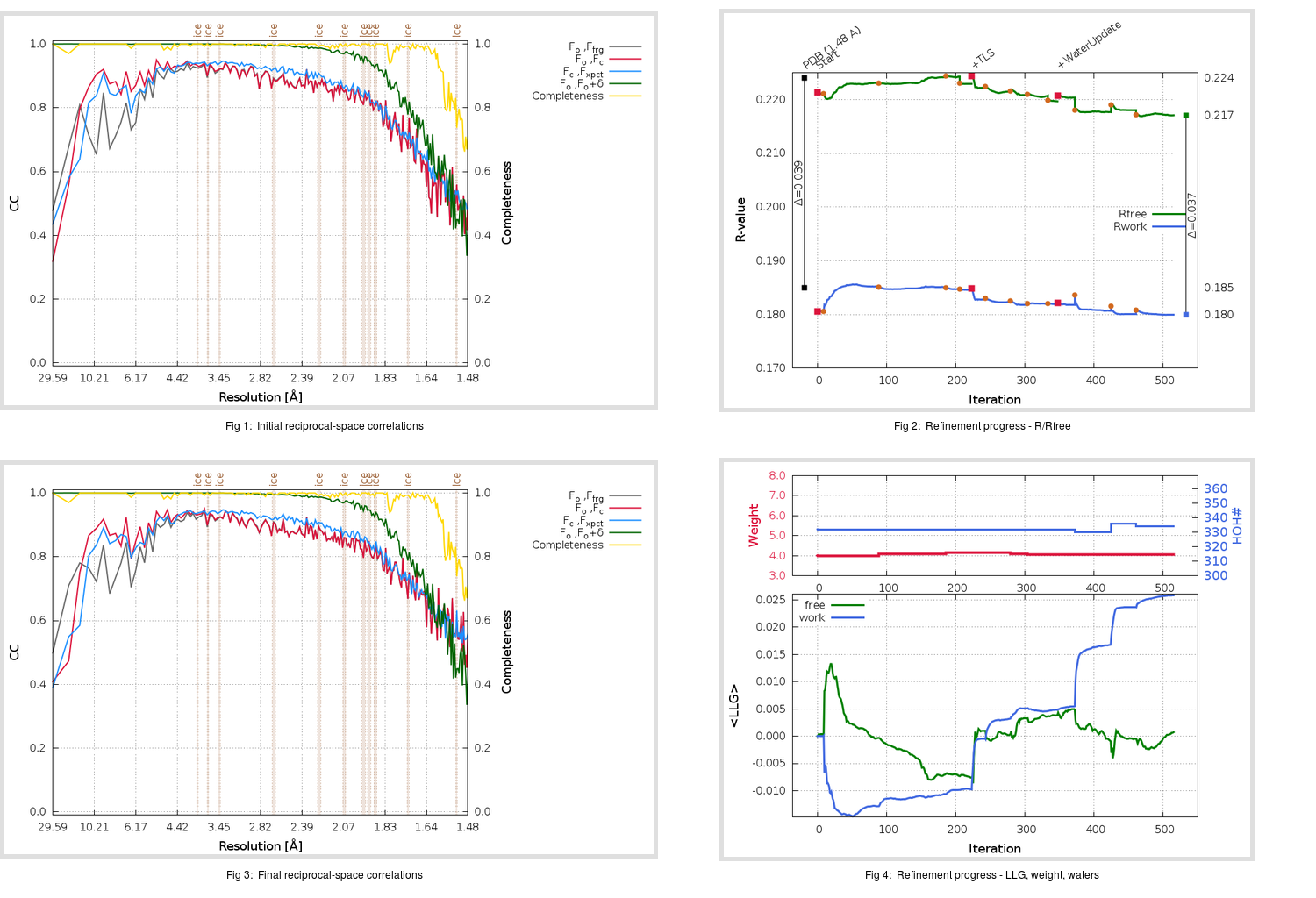Content:

## Deposited

` `
 Date deposited Date data collection Resolution R, Rfree 20200315 20200305 1.48 0.1830 0.2240

Molprobity (CCP4 7.0 version) summary:

```Ramachandran outliers =   0.33 %
favored =  98.01 %
Rotamer outliers      =   1.52 %
C-beta deviations     =     0
Clashscore            =   5.29
RMS(bonds)            =   0.0134
RMS(angles)           =   1.84
MolProbity score      =   1.42
Resolution            =   1.48
R-work                =   0.1830
R-free                =   0.2240
```

```Number of waters      =   332

<B> (all atoms) =   24.18 ( sd =    9.58 ) for       2724 non-hydrogen atoms
<B>   (protein) =   22.64 ( sd =    8.59 ) for       2370 non-hydrogen atoms
<B>     (water) =   34.86 ( sd =    9.36 ) for        332 non-hydrogen atoms
<B>    (others) =   27.41 ( sd =   10.26 ) for         22 non-hydrogen atoms

B min/max       (all non-hydrogen atoms) =   11.94 /   71.05
B min/max   (protein non-hydrogen atoms) =   11.94 /   64.56
B min/max     (water non-hydrogen atoms) =   13.12 /   71.05
B min/max     (other non-hydrogen atoms) =   20.44 /   34.65
```

## BUSTER (re-)refinement

` `

Molprobity (CCP4 7.0 version) summary:

```Ramachandran outliers =   0.33 %
favored =  98.68 %
Rotamer outliers      =   1.89 %
C-beta deviations     =     0
Clashscore            =   2.54
RMS(bonds)            =   0.0113
RMS(angles)           =   1.57
MolProbity score      =   1.25
Resolution            =   1.48
R-work                =   0.1800
R-free                =   0.2171
```

```Number of waters      =   334

<B> (all atoms) =   25.30 ( sd =    8.97 ) for       2726 non-hydrogen atoms
<B>   (protein) =   23.59 ( sd =    7.45 ) for       2370 non-hydrogen atoms
<B>     (water) =   37.05 ( sd =    9.81 ) for        334 non-hydrogen atoms
<B>    (others) =   30.21 ( sd =   10.14 ) for         22 non-hydrogen atoms

B min/max       (all non-hydrogen atoms) =   13.72 /   64.12
B min/max   (protein non-hydrogen atoms) =   13.72 /   64.12
B min/max     (water non-hydrogen atoms) =   14.89 /   63.55
B min/max     (other non-hydrogen atoms) =   25.50 /   34.58
```

Refinement progression:Results:

` `
 File Remark 5RFB_aB_refine.01_03_refine.pdb.gz exact refinement commands are in header 5RFB_aB_refine.01_03_refine.mtz.gz including original deposited data and several re-refinement map coefficients 5RFB_aB_refine.01_03_BUSTER_model.cif.gz including any non-standard compound restraints 5RFB_aB_refine.01_03_BUSTER_refln.cif.gz